International
Tables for
Crystallography
Volume B
Reciprocal space
Edited by U. Shmueli

International Tables for Crystallography (2006). Vol. B, ch. 1.3, p. 70   | 1 | 2 |

## Section 1.3.4.2.2.9. Convolution theorems with crystallographic symmetry

G. Bricognea

aMRC Laboratory of Molecular Biology, Hills Road, Cambridge CB2 2QH, England, and LURE, Bâtiment 209D, Université Paris-Sud, 91405 Orsay, France

#### 1.3.4.2.2.9. Convolution theorems with crystallographic symmetry

| top | pdf |

The standard convolution theorems derived in the absence of symmetry are readily seen to follow from simple properties of functions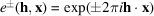(denoted simply e in formulae which are valid for both signs), namely: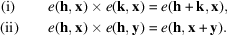These relations imply that the families of functions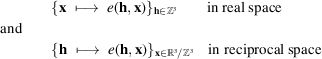both generate an algebra of functions, i.e. a vector space endowed with an internal multiplication, since (i) and (ii) show how to linearize products'.

Friedel's law (when applicable) on the one hand, and the Fourier relation between intensities and the Patterson function on the other hand, both follow from the property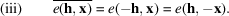When crystallographic symmetry is present, the convolution theorems remain valid in their original form if written out in terms of expanded' data, but acquire a different form when rewritten in terms of symmetry-unique data only. This rewriting is made possible by the extra relation (Section 1.3.4.2.2.5)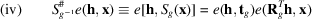or equivalently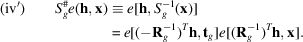The kernels of symmetrized Fourier transforms are not the functions e but rather the symmetrized sums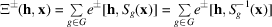for which the linearization formulae are readily obtained using (i), (ii) and (iv) as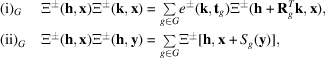where the choice of sign in ± must be the same throughout each formula.

Formulae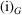defining the `structure-factor algebra' associated to G were derived by Bertaut (1955c, 1956b,c, 1959a,b) and Bertaut & Waser (1957)in another context.

The forward convolution theorem (in discrete form) then follows. Let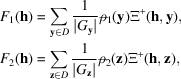then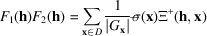with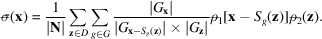The backward convolution theorem is derived similarly. Let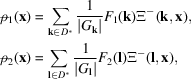then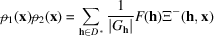with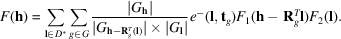Both formulae are simply orbit decompositions of their symmetry-free counterparts.

### References

Bertaut, E. F. (1955c). Fonction de répartition: application à l'approache directe des structures. Acta Cryst. 8, 823–832.Google Scholar
Bertaut, E. F. (1956b). Tables de linéarisation des produits et puissances des facteurs de structure. Acta Cryst. 9, 322–323.Google Scholar
Bertaut, E. F. (1956c). Algèbre des facteurs de structure. Acta Cryst. 9, 769–770.Google Scholar
Bertaut, E. F. (1959a). IV. Algèbre des facteurs de structure. Acta Cryst. 12, 541–549.Google Scholar
Bertaut, E. F. (1959b). V. Algèbre des facteurs de structure. Acta Cryst. 12, 570–574.Google Scholar
Bertaut, E. F. & Waser, J. (1957). Structure factor algebra. II. Acta Cryst. 10, 606–607.Google Scholar